Chopped & Diced Vegetables

Chopped & Diced Vegetables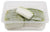Rs. 22.00
Rs. 39.75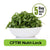Rs. 18.00
Rs. 25.75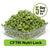Rs. 28.00
Rs. 42.00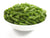Rs. 27.00
Rs. 42.00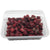Rs. 24.00
Rs. 32.75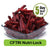Rs. 30.00
Rs. 35.75
Sold Out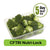Rs. 149.00
Rs. 211.00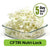Rs. 27.00
Rs. 38.00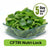Rs. 29.00
Rs. 45.00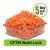Rs. 25.00
Rs. 62.00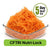Rs. 45.00
Rs. 70.00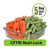Rs. 58.00
Rs. 80.00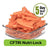Rs. 45.00
Rs. 70.00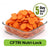Rs. 45.00
Rs. 70.00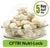Rs. 65.00
Rs. 76.00Rs. 28.00
Rs. 37.50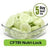Rs. 29.00
Rs. 42.00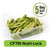Rs. 27.00
Rs. 35.25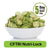Rs. 27.00
Rs. 36.25
Sold Out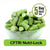Rs. 34.00
Rs. 56.00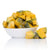Rs. 34.00
Rs. 50.50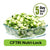Rs. 45.00
Rs. 65.00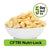Rs. 49.00
Rs. 61.00